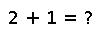# cholfact(A)

cholfact(A; shift=0, perm=Int[]) -> CHOLMOD.Factor

Compute the Cholesky factorization of a sparse positive definite matrix `A`. A fill-reducing permutation is used. `F = cholfact(A)` is most frequently used to solve systems of equations with `F\b`, but also the methods `diag`, `det`, `logdet` are defined for `F`. You can also extract individual factors from `F`, using `F[:L]`. However, since pivoting is on by default, the factorization is internally represented as `A == P'*L*L'*P` with a permutation matrix `P`; using just `L` without accounting for `P` will give incorrect answers. To include the effects of permutation, it's typically preferable to extact "combined" factors like `PtL = F[:PtL]` (the equivalent of `P'*L`) and `LtP = F[:UP]` (the equivalent of `L'*P`).

Setting optional `shift` keyword argument computes the factorization of `A+shift*I` instead of `A`. If the `perm` argument is nonempty, it should be a permutation of `1:size(A,1)` giving the ordering to use (instead of CHOLMOD's default AMD ordering).

The function calls the C library CHOLMOD and many other functions from the library are wrapped but not exported.

## Examples

Checking you are not a robot: